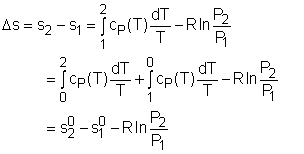Ch 6. Entropy Multimedia Engineering Thermodynamics Entropy Tds Relations EntropyChange IsentropicProcess IsentropicEfficiency EntropyBalance (1) EntropyBalance (2) ReversibleWork
 Chapter 1. Basics 2. Pure Substances 3. First Law 4. Energy Analysis 5. Second Law 6. Entropy 7. Exergy Analysis 8. Gas Power Cyc 9. Brayton Cycle 10. Rankine Cycle Appendix Basic Math Units Thermo Tables Search eBooks Dynamics Fluids Math Mechanics Statics Thermodynamics Author(s): Meirong Huang Kurt Gramoll ©Kurt GramollTHERMODYNAMICS - THEORY

Entropy Change of Pure Substances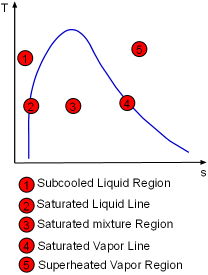The Entropy of a Pure Substance Determined by Tables

The entropy of a pure substance is determined from tables in the same manner as other properties such as v, u, and h. The value of entropy at a specified state is determined just like any other property.

• Compressed liquid: from tables
• Saturated liquid (sf): from tables
• Saturated mixture: s = sf + xsfg
where
x = quality
sfg = sg - sf
• Saturated vapor (sg): from tables
• Superheated vapor: from tables

If the compressed liquid data are not available, the entropy of the compressed liquid can be approximated by the saturated liquid at the same temperature.

scompressed@T,P = sf@T

 Saturated Water Temperature Table Saturated Water Pressure Table Superheated Steam Table
Water Tables

Entropy Change of Solids and Liquids

For an incompressible substances, the specific volume does not change during a process. That is,

dv = 0

du = c(T) dT

Most solids and liquids can be approximated as incompressible substances. The entropy change of solids and liquids can be obtained by applying the above two equations to the first Tds equation.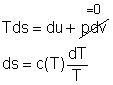The entropy change during a process 1-2 is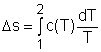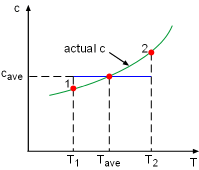Constant Specific Heat Used in Small Temperature Interval

If the temperature variation (T2 - T1) is small over the entire process, an average specific heat (cave) is used and treated as a constant (constant-specific-heats assumption). Then the entropy change can be evaluated byEntropy Change of Ideal Gases

The property relations for ideal gases are:

pv = RT
du = cvdT
dh = cPdT

The first relation of entropy change for ideal gases is obtained by replacing P by RT/v and du by cvdT in the first Tds equation:

Tds = du + Pdv
= cvdT + RTdv/v

ds = cvdT/T + Rdv/v

Integrating ds from state 1 to state 2 gives the first relation of entropy change for ideal gases.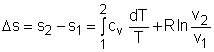The second relation of entropy change for ideal gases is obtained by replacing v by RT/P and dh by cPdT in the second Tds equation:

Tds = dh - vdP
= cPdT - RTdP/P

ds = cPdT/T - RdP/P

Integrating ds from state 1 to state 2 gives the second relation of entropy change for ideal gases.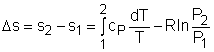A common approximation is made by assuming that the specific heats of ideal gases are constants if the temperature variation is small (constant-specific-heats assumption). Replacing cv and cP with constants cv,av and cP,av in the first and second relations of entropy change and integrating from state 1 to state 2 gives,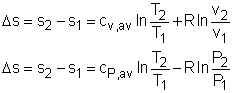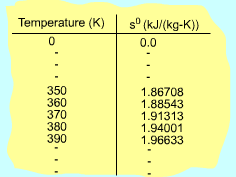Function s0 is a Function of
Temperature only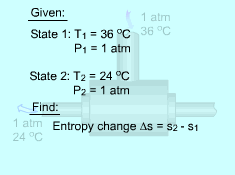Approximate Analysis and
Exact Analysis of Entropy Change
during a Cooling Process

If the temperature variation is large, the variation of specific heats with temperature should be considered and relations between cv(T), cP(T) and temperature are needed to integrate the first and second relations. For convenience, a function s0, standard-state entropy, is defined as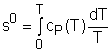s0 is a function of temperature only and is tabulated with temperature. Absolute zero is used as the reference temperature.

Replacing T as T1 and T2 in the above definition gives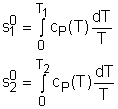Then the entropy change of ideal gases during a process 1-2 is